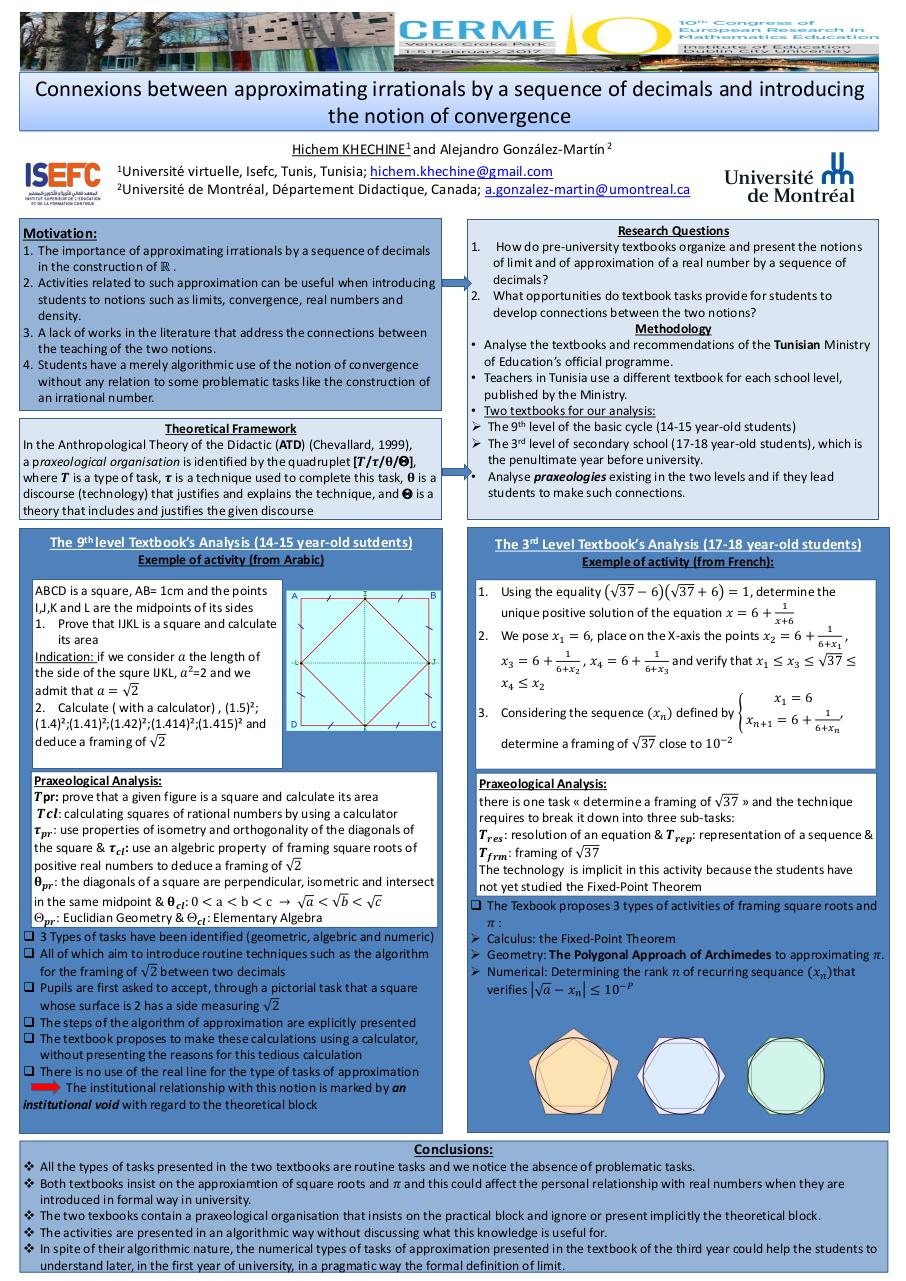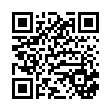# poster Cerme 2 .pdf

### File information

Original filename: poster Cerme 2.pdf
Author: DELL 3520 DUAL CORE

This PDF 1.5 document has been generated by MicrosoftÂ® PowerPointÂ® 2010, and has been sent on pdf-archive.com on 25/03/2017 at 12:09, from IP address 41.225.x.x. The current document download page has been viewed 416 times.
File size: 1.1 MB (1 page).
Privacy: public file

poster Cerme 2.pdf (PDF, 1.1 MB)

### Document preview

Connexions between approximating irrationals by a sequence of decimals and introducing
the notion of convergence
Hichem KHECHINE1 and Alejandro González-Martín 2
1Université virtuelle, Isefc, Tunis, Tunisia; hichem.khechine@gmail.com
2Université de Montréal, Département Didactique, Canada; a.gonzalez-martin@umontreal.ca
Motivation:
1. The importance of approximating irrationals by a sequence of decimals
in the construction of ℝ .
2. Activities related to such approximation can be useful when introducing
students to notions such as limits, convergence, real numbers and
density.
3. A lack of works in the literature that address the connections between
the teaching of the two notions.
4. Students have a merely algorithmic use of the notion of convergence
without any relation to some problematic tasks like the construction of
an irrational number.
Theoretical Framework
In the Anthropological Theory of the Didactic (ATD) (Chevallard, 1999),
a praxeological organisation is identified by the quadruplet [𝑻/𝝉/𝛉/],
where 𝑻 is a type of task, 𝝉 is a technique used to complete this task, 𝛉 is a
discourse (technology) that justifies and explains the technique, and  is a
theory that includes and justifies the given discourse

Research Questions
1. How do pre-university textbooks organize and present the notions
of limit and of approximation of a real number by a sequence of
decimals?
2. What opportunities do textbook tasks provide for students to
develop connections between the two notions?
Methodology
• Analyse the textbooks and recommendations of the Tunisian Ministry
of Education’s official programme.
• Teachers in Tunisia use a different textbook for each school level,
• Two textbooks for our analysis:
 The 9th level of the basic cycle (14-15 year-old students)
 The 3rd level of secondary school (17-18 year-old students), which is
the penultimate year before university.
• Analyse praxeologies existing in the two levels and if they lead
students to make such connections.

The 9th level Textbook’s Analysis (14-15 year-old sutdents)

The 3rd Level Textbook’s Analysis (17-18 year-old students)

Exemple of activity (from Arabic)

Exemple of activity (from French):

ABCD is a square, AB= 1cm and the points
I,J,K and L are the midpoints of its sides
1. Prove that IJKL is a square and calculate
its area
Indication: if we consider 𝑎 the length of
the side of the squre IJKL, 𝑎²=2 and we
2. Calculate ( with a calculator) , (1.5)²;
(1.4)²;(1.41)²;(1.42)²;(1.414)²;(1.415)² and
deduce a framing of 2

1. Using the equality

37 − 6

37 + 6 = 1, determine the

unique positive solution of the equation 𝑥 = 6 +

1
𝑥+6

2. We pose 𝑥1 = 6, place on the X-axis the points 𝑥2 = 6 +
𝑥3 = 6 +

1
6+𝑥2

, 𝑥4 = 6 +

1
6+𝑥3

1
6+𝑥1

,

and verify that 𝑥1 ≤ 𝑥3 ≤ 37 ≤

𝑥4 ≤ 𝑥2
3. Considering the sequence (𝑥𝑛 ) defined by

𝑥1 = 6
𝑥𝑛+1 = 6 +

1 ,
6+𝑥𝑛

determine a framing of 37 close to 10−2

Praxeological Analysis:
𝑻pr: prove that a given figure is a square and calculate its area
𝑻𝒄𝒍: calculating squares of rational numbers by using a calculator
𝝉𝒑𝒓 : use properties of isometry and orthogonality of the diagonals of
the square &amp; 𝝉𝒄𝒍 : use an algebric property of framing square roots of
positive real numbers to deduce a framing of 2
𝛉𝒑𝒓 : the diagonals of a square are perpendicular, isometric and intersect
in the same midpoint &amp; 𝛉𝒄𝒍 : 0 &lt; a &lt; b &lt; c → 𝑎 &lt; 𝑏 &lt; 𝑐
𝒑𝒓 : Euclidian Geometry &amp; 𝒄𝒍 : Elementary Algebra
 3 Types of tasks have been identified (geometric, algebric and numeric)
 All of which aim to introduce routine techniques such as the algorithm
for the framing of 2 between two decimals
 Pupils are first asked to accept, through a pictorial task that a square
whose surface is 2 has a side measuring 2
 The steps of the algorithm of approximation are explicitly presented
 The textbook proposes to make these calculations using a calculator,
without presenting the reasons for this tedious calculation
 There is no use of the real line for the type of tasks of approximation
The institutional relationship with this notion is marked by an
institutional void with regard to the theoretical block

Praxeological Analysis:
there is one task « determine a framing of 37 » and the technique
requires to break it down into three sub-tasks:
𝑻𝒓𝒆𝒔 : resolution of an equation &amp; 𝑻𝒓𝒆𝒑 : representation of a sequence &amp;
𝑻𝒇𝒓𝒎 : framing of 37
The technology is implicit in this activity because the students have
not yet studied the Fixed-Point Theorem
 The Texbook proposes 3 types of activities of framing square roots and
𝜋:
 Calculus: the Fixed-Point Theorem
 Geometry: The Polygonal Approach of Archimedes to approximating 𝜋.
 Numerical: Determining the rank 𝑛 of recurring sequance (𝑥𝑛 )that
−𝑃
verifies 𝑎 − 𝑥𝑛 ≤ 10

Conclusions:
 All the types of tasks presented in the two textbooks are routine tasks and we notice the absence of problematic tasks.
 Both textbooks insist on the approxiamtion of square roots and 𝜋 and this could affect the personal relationship with real numbers when they are
introduced in formal way in university.
 The two texbooks contain a praxeological organisation that insists on the practical block and ignore or present implicitly the theoretical block.
 The activities are presented in an algorithmic way without discussing what this knowledge is useful for.
 In spite of their algorithmic nature, the numerical types of tasks of approximation presented in the textbook of the third year could help the students to
understand later, in the first year of university, in a pragmatic way the formal definition of limit.#### HTML Code

Copy the following HTML code to share your document on a Website or Blog

#### QR Code### Related keywords